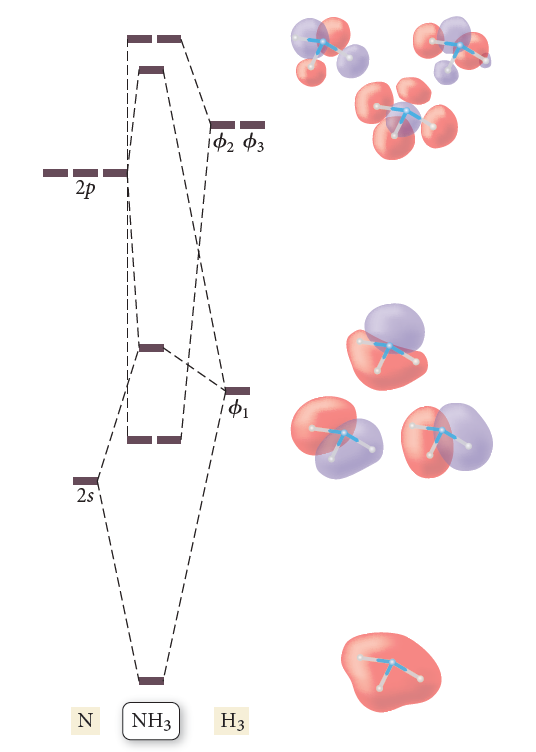# Problem: The results of a molecular orbital calculation for NH3 are shown here. Examine each of the orbitals and classify them as bonding, antibonding, or nonbonding. Assign the correct number of electrons to the energy diagram. According to this energy diagram, is NH3 stable? Explain.

###### FREE Expert Solution

We’re being asked to examine each of the orbitals and classify them as bonding, antibonding, or nonbonding then assign the correct number of electrons to the energy diagram. Lastly, examine if NH3 is stable using the info gathered.

Step 1: The total number of valence electrons present in NH3 is:

Group             Valence Electrons

H         1A                   3 × 1 e3 e

N         5A                  1 × 5 e5 e

Total:  8 valence e

92% (108 ratings)###### Problem Details

The results of a molecular orbital calculation for NH3 are shown here. Examine each of the orbitals and classify them as bonding, antibonding, or nonbonding. Assign the correct number of electrons to the energy diagram. According to this energy diagram, is NH3 stable? Explain.Frequently Asked Questions

What scientific concept do you need to know in order to solve this problem?

Our tutors have indicated that to solve this problem you will need to apply the MO Theory: Bond Order concept. You can view video lessons to learn MO Theory: Bond Order. Or if you need more MO Theory: Bond Order practice, you can also practice MO Theory: Bond Order practice problems.

What professor is this problem relevant for?

Based on our data, we think this problem is relevant for Professor Khatiwada's class at Abraham Baldwin Agricultural College.